# Training a Pytorch Classic MNIST GAN on Google Colab

Marton Trencseni - Tue 02 March 2021 - Machine Learning

## Introduction

Generative Adversarial Networks (GANs) were introduced by Ian Goodfellow in 2014. GANs are able to learn a probability distribution and generate new samples from noise per the probability distribution. In plain english, this means GANs can be trained on a set of images (or audio, etc), and will be able to produce realistic looking new "fake" images (or audio, etc). To connect the two explanations: MNIST digits are 28x28 grascale images and pixel values are 0-255, so an MNIST digit can be thought of as a 28x28=784 element vector, where each element is 0-255. Some (tiny) portions of this space look like digits, most parts look like noise (also others look like letters, etc.)

For another explanation, here is Wikipedia:

A generative adversarial network (GAN) is a class of machine learning frameworks designed by Ian Goodfellow and his colleagues in 2014. Two neural networks contest with each other in a game (in the form of a zero-sum game, where one agent's gain is another agent's loss). Given a training set, this technique learns to generate new data with the same statistics as the training set. For example, a GAN trained on photographs can generate new photographs that look at least superficially authentic to human observers, having many realistic characteristics. Though originally proposed as a form of generative model for unsupervised learning, GANs have also proven useful for semi-supervised learning, fully supervised learning, and reinforcement learning. The core idea of a GAN is based on the "indirect" training through the discriminator, which itself is also being updated dynamically. This basically means that the generator is not trained to minimize the distance to a specific image, but rather to fool the discriminator. This enables the model to learn in an unsupervised manner.

I took an old implementation from the abandoned Pytorch-GAN repo, and made it work on Google Colab, the notebook is here. You can just run the notebook and generate your own digits.

Note: In a previous post, I tried to train the Softmax MNIST GAN in Pytorch Lighting. I decided to stop using Pytorch Lightning for now, because I ran into numerous framework issues, see this and this issue I opened. Also, the Softmax GAN itself gave me trouble even on Pytorch, so I decided to take a step back and start with Goodfellow's classic GAN.

## Architecture

Conceptually a GAN program has 6 parts:

1. Data we're trying to generate
2. Discriminator network
3. Generator network
4. Discriminator's loss function
5. Generator's loss function
6. Training process

The explanation of the role of the generator and discriminator networks, from Wikipedia:

The generative network generates candidates while the discriminative network evaluates them. The contest operates in terms of data distributions. Typically, the generative network learns to map from a latent space to a data distribution of interest, while the discriminative network distinguishes candidates produced by the generator from the true data distribution. The generative network's training objective is to increase the error rate of the discriminative network (i.e., "fool" the discriminator network by producing novel candidates that the discriminator thinks are not synthesized (are part of the true data distribution)). A known dataset serves as the initial training data for the discriminator. Training it involves presenting it with samples from the training dataset, until it achieves acceptable accuracy. The generator trains based on whether it succeeds in fooling the discriminator. Typically the generator is seeded with randomized input that is sampled from a predefined latent space (e.g. a multivariate normal distribution). Thereafter, candidates synthesized by the generator are evaluated by the discriminator. Independent backpropagation procedures are applied to both networks so that the generator produces better images, while the discriminator becomes more skilled at flagging synthetic images. The generator is typically a deconvolutional neural network, and the discriminator is a convolutional neural network.

For this blog post:

1. Data = MNIST digits.
2. Discriminator = a convolutional neural network (CNN), which given an MNIST digits, tries to distinguish real (from the training set) and generated (by the generator) images. More precisely, it predicts the probability of the digit being real.
3. Generator = a deconvolutional network, which given some random input, generates a 28x28 image; once trained, the generator's output should be MNIST-like.

Note that 1-3. above is the same for many MNIST GAN architectures, whether it's Classic, Softmax, Wasserstein, etc. For the Classic GAN, we use Binary Cross Entropy (BCE) in both the generator and discriminator's loss function, ie. a measure of the distance between two probability distributions:

1. Discriminator's loss function = BCE between the discriminator's predicted probabilities and the digit's ground truth (0 for generated or 1 for real from the MNIST dataset), computed over an even mix of real and fake.
2. Generator's loss function = BCE between the discriminator's prediction over generated images and the constant 1.
3. Training process = one epoch is going through all 60K 28x28 MNIST digits, and for each one also generating a fake digit, in batches of 64. Use Stochastic gradient descent (Adam) to update the network weights on each batch based on the losses.

## Code

First, let's see the Pytorch code the discriminator, a straighforward CNN:

class Discriminator(nn.Module):
def __init__(self):
super(Discriminator, self).__init__()
self.model = nn.Sequential(
nn.Linear(int(np.prod(img_shape)), 512),
nn.LeakyReLU(0.2, inplace=True),
nn.Linear(512, 256),
nn.LeakyReLU(0.2, inplace=True),
nn.Linear(256, 1),
nn.Sigmoid(),
)
def forward(self, img):
img_flat = img.view(img.size(0), -1)
validity = self.model(img_flat)
return validity


Note that "straighforward" is a professional euphemism. One of the reasons Deep Learning took off a few years ago is that people realized that introducing non-linearities like ReLUs makes the network work (vs. purely linear NNs). Guessing what neural network works for a given use-case is a mix of black art, experimentation and copy/paste from existing.

The generator:

class Generator(nn.Module):
def __init__(self):
super(Generator, self).__init__()
def block(in_feat, out_feat, normalize=True):
layers = [nn.Linear(in_feat, out_feat)]
if normalize:
layers.append(nn.BatchNorm1d(out_feat, 0.8))
layers.append(nn.LeakyReLU(0.2, inplace=True))
return layers
self.model = nn.Sequential(
*block(opt.latent_dim, 128, normalize=False),
*block(128, 256),
*block(256, 512),
*block(512, 1024),
nn.Linear(1024, int(np.prod(img_shape))),
nn.Tanh()
)
def forward(self, z):
img = self.model(z)
img = img.view(img.size(0), *img_shape)
return img


As mentioned above, the discriminator's loss is binary cross entropy (BCE), which is BCELoss in Pytorch:

bce_loss = torch.nn.BCELoss()
...
generator_loss = bce_loss(discriminator(gen_imgs), real)
...
real_loss = bce_loss(discriminator(real_imgs),         real)
fake_loss = bce_loss(discriminator(gen_imgs.detach()), fake)
discriminator_loss = (real_loss + fake_loss) / 2


The overall logic of the training loop is (see comments):

real = Variable(Tensor(imgs.size(0), 1).fill_(1.0), requires_grad=False)
real_imgs = Variable(imgs.type(Tensor))
#  train Generator
# sample noise as generator input
z = Variable(Tensor(np.random.normal(0, 1, (imgs.shape, opt.latent_dim))))
# generate a batch of images
gen_imgs = generator(z)
# loss measures generator's ability to fool the discriminator:
# for the generated images, the generator wants the discriminator to think they're real (1)
# so if the discriminator(gen_imgs) == real == 1, then the generator is doing a good job, there is no loss
generator_loss = bce_loss(discriminator(gen_imgs), real)
generator_loss.backward()
generator_optimizer.step()
# train Discriminator
# loss measure discriminator's ability to classify real from generated samples:
real_loss = bce_loss(discriminator(real_imgs),         real)
fake_loss = bce_loss(discriminator(gen_imgs.detach()), fake)
discriminator_loss = (real_loss + fake_loss) / 2
discriminator_loss.backward()
discriminator_optimizer.step()


## Results

Note that this is the first GAN invented, so it still suffers from childhood issues such as "mode collapse", where the GAN mostly emits the same digits. A better name would be "range collapse" in my opinion, in mathematics range is the term for the space that a function generates into.

Below are samples of MNIST digits produced by the GAN after 1, 5, 10, 50, 100, 150 and 200 epochs of training (an epoch is a full go through the MNIST dataset, consisting of 60K digits):

Epoch 1: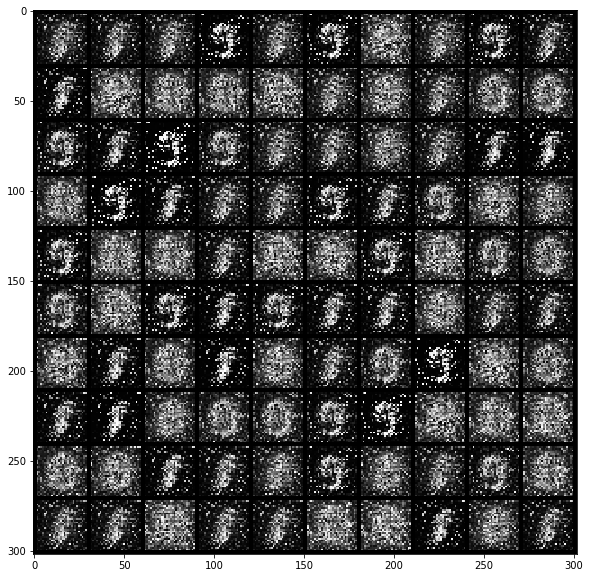Epoch 5: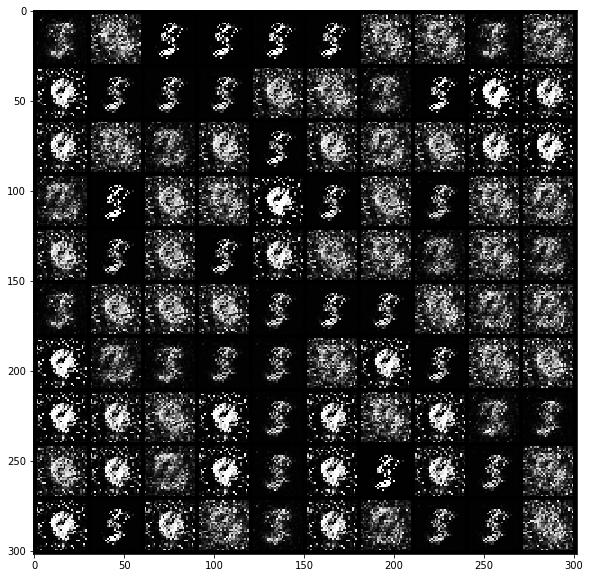Epoch 10: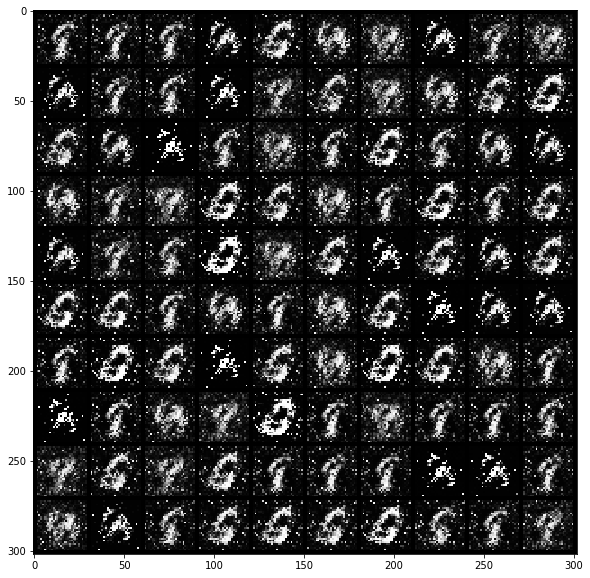Epoch 50: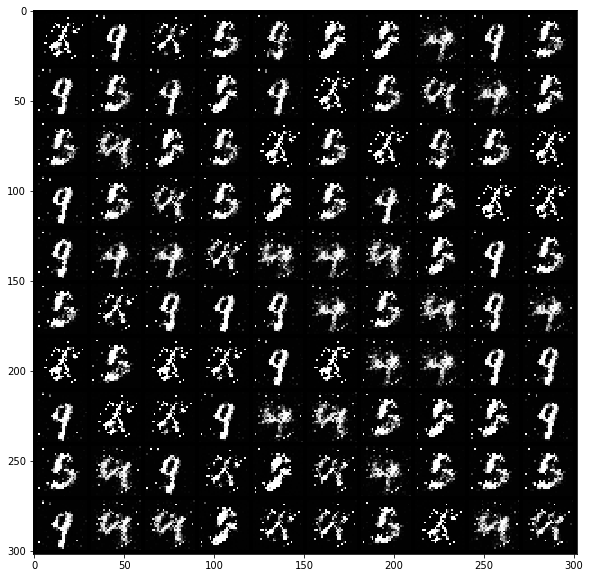Epoch 100: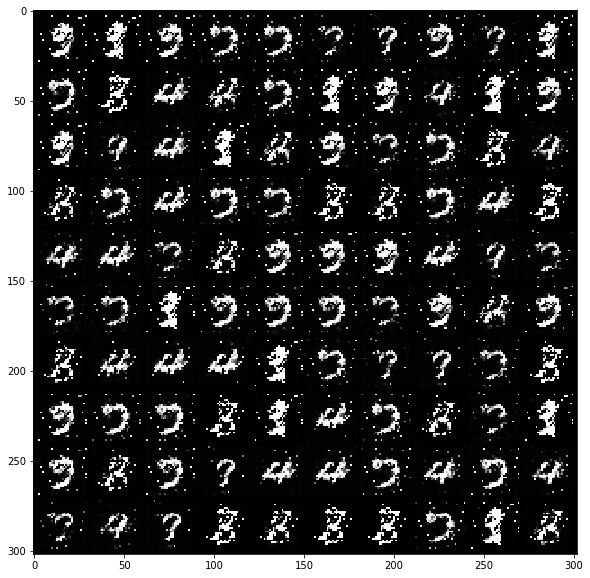Epoch 150: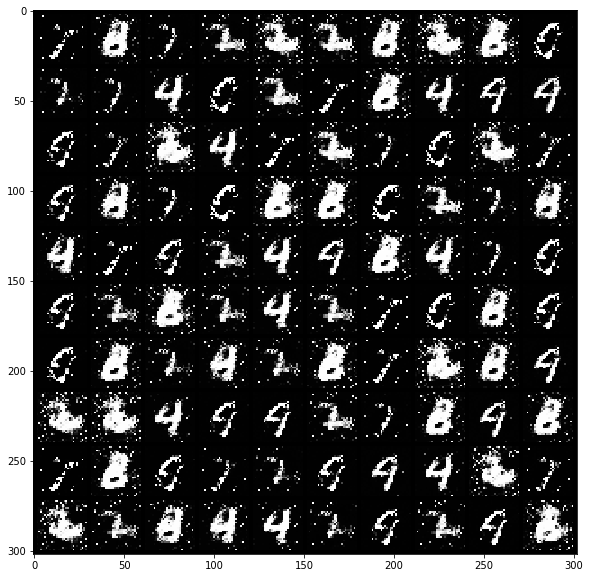Epoch 200: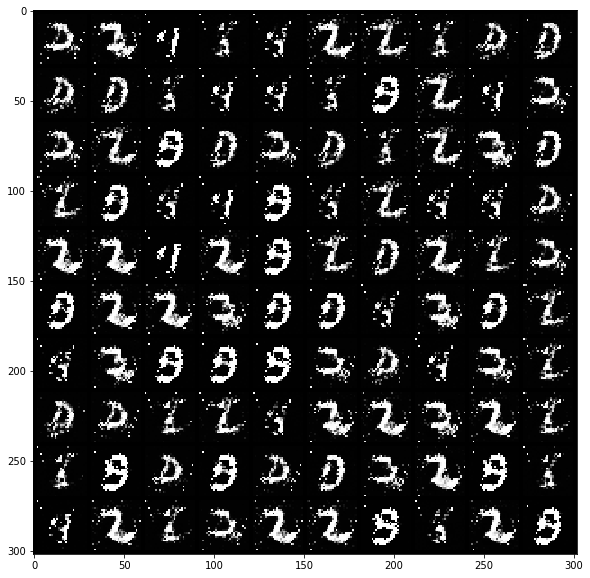## Conclusion

1. The digits above look okay, many of them are recognizable, but overall the quality is not great.
2. The network suffers from range collapse, mostly it's trying to generate 2s and 8s.

In the next post, I will look at the Wasserstein GAN, which doesn't suffer from range collapse and produces beautiful MNIST digits.